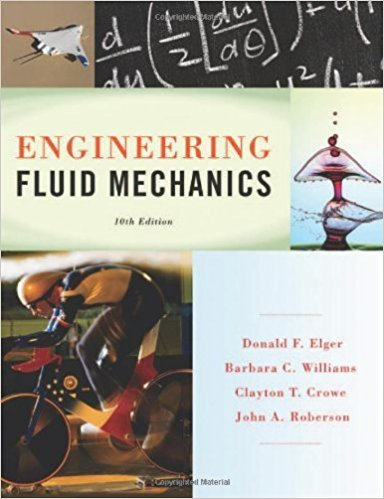×
×

# A water bug is suspended on the surface of a pond by surface tension (water does not wetISBN: 9781118164297 288

## Solution for problem 2.59 Chapter 2

Engineering Fluid Mechanics | 10th Edition

• Textbook Solutions
• 2901 Step-by-step solutions solved by professors and subject experts
• Get 24/7 help from StudySoup virtual teaching assistantsEngineering Fluid Mechanics | 10th Edition

4 5 1 360 Reviews
29
2
Problem 2.59

A water bug is suspended on the surface of a pond by surface tension (water does not wet the legs). The bug has six legs, and each leg is in contact with the water over a length of 5 mm. What is the maximum mass (in grams) of the bug if it is to avoid sinking?

Step-by-Step Solution:
Step 1 of 3

Week 5 Physics 2 for Engineering Chapter 24: Capacitance and Dielectrics Capacitors store charge C = Q/V Unit is Farads, 1 Farad = 1 C/V Review: Q = σ A V = Ed Parallel Plate Capacitor C = A Ɛ / 0 C = Q/V = σ A/ Ed = A Ɛ / d 0 Capacitors in series Charge on each capacitor is the same Voltage on each...

Step 2 of 3

Step 3 of 3

##### ISBN: 9781118164297

Unlock Textbook Solution